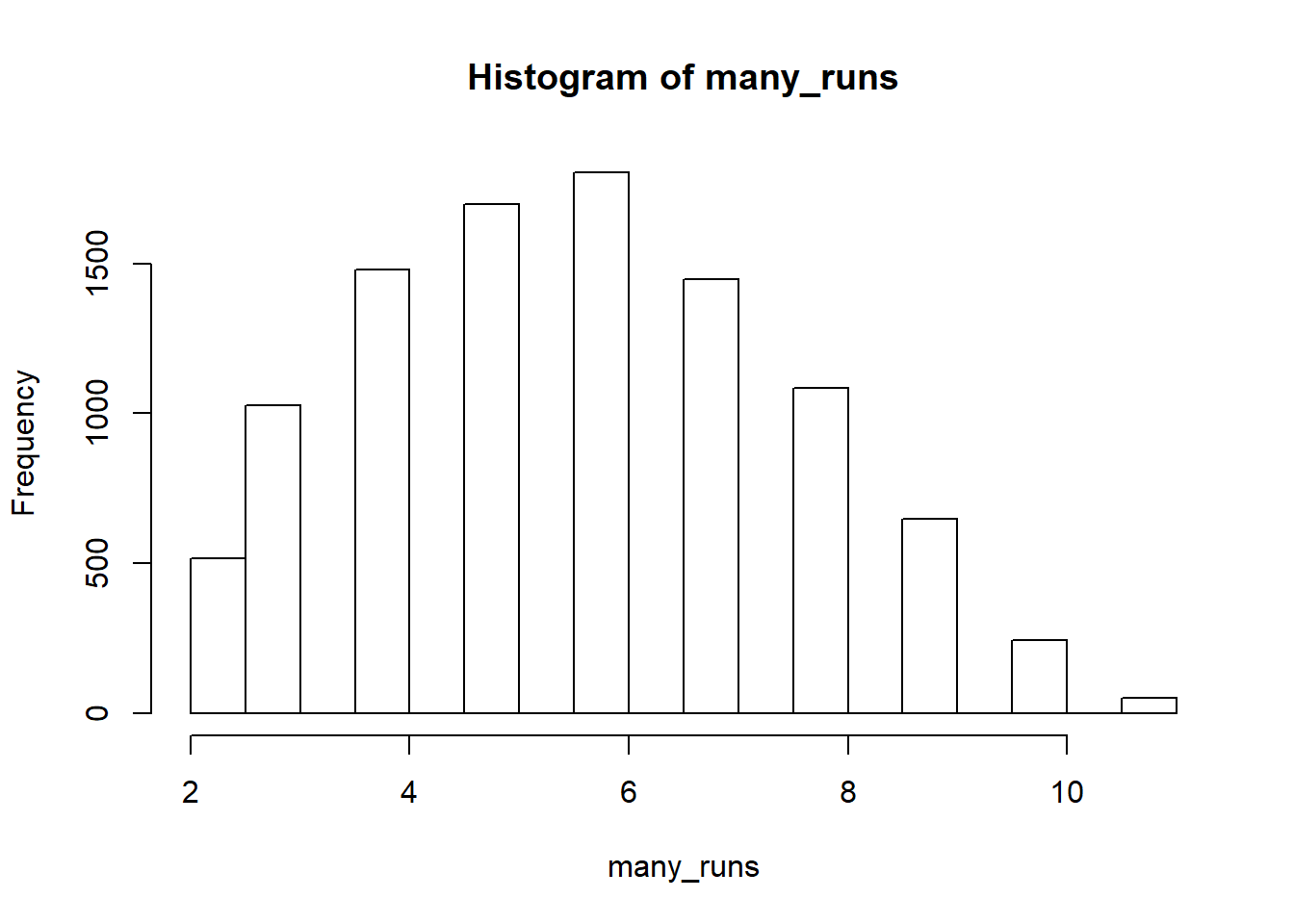# How Many Socks Until You Get a Match?

## Intro

Here’s another FiveThirtyEight puzzle from The Riddler. This post is an answer to the Riddler Classic https://fivethirtyeight.com/features/can-you-find-a-matching-pair-of-socks/.

### Question

I have 10 pairs of socks in a drawer. Each pair is distinct from another and consists of two matching socks. Alas, I’m negligent when it comes to folding my laundry, and so the socks are not folded into pairs. This morning, fumbling around in the dark, I pull the socks out of the drawer, randomly and one at a time, until I have a matching pair of socks among the ones I’ve removed from the drawer.

On average, how many socks will I pull out of the drawer in order to get my first matching pair?

With 10 pairs of socks, I can get a pair in 5.68 steps, on average. Here’s how we can simulate the results.

## Simulation in R

#### Load the tidyverse and parallel packages

``````library(tidyverse)
## -- Attaching packages ------------------- tidyverse 1.3.0 --
## v ggplot2 3.2.1     v purrr   0.3.3
## v tibble  2.1.3     v dplyr   0.8.3
## v tidyr   1.0.0     v stringr 1.4.0
## v readr   1.3.1     v forcats 0.4.0
## -- Conflicts ---------------------- tidyverse_conflicts() --
library(foreach)
##
## Attaching package: 'foreach'
## The following objects are masked from 'package:purrr':
##
##     accumulate, when
library(doSNOW)

### Create clusters

The way these packages handle parallel computing is to create clusters that can initiate an R script. Each cluster then uses a core to run the code. This is the standard way to initialize and register the number of clusters.

``````cl<-makeCluster(3) # change the 3 to the number of CPU cores you want to use
registerDoSNOW(cl)``````

### Foreach loop

Just like the other Riddler, I’m going to write a foreach-loop. Here’s a link about the basics of `foreach` https://www.r-bloggers.com/parallel-r-loops-for-windows-and-linux/.

`foreach` usually results in a list, but we can get it to output a vector by adding `.combine = 'c'`. `%dopar%` is the parallel version of `%do%`, and the only thing different is that it requires preregistered clusters.

Every element of the `many_runs` vector will be the number of socks required to get a match.

``````# Initialize starting variables

socks = 1:10
number_of_matches = 1e4

# Run simulations

many_runs = foreach(1:number_of_matches, .combine = 'c') %dopar% {

# Two sock elements because there are pairs in the drawer
drawer = c(socks,socks)

# Start empty handed
pull_sock = NULL
socks_in_hand = NULL

# Run the while loop until there's one duplicate and length(unique) is shorter than length
while ( length(unique(socks_in_hand)) == length(socks_in_hand) ) {

# sample and do not replace
pull_sock = sample(drawer, 1, replace = FALSE)

# put the sock in hand
socks_in_hand = c(socks_in_hand, pull_sock)

# remove the sock from the drawer
#  The match returns the first matching index of the pull sock in the drawer
#  - removes that index
# So the new drawer is the old drawer without the pulled sock
drawer = drawer[-match(pull_sock, drawer)]

}

# send this number to the many_runs vector
length(socks_in_hand)
}``````

### Stop clusters

Now we’ll close our clusters. Eventually, the clusters close after some period of inactivity, but it’s best practice to stop them in the code.

``stopCluster(cl)``

## Results

``kableExtra::kable(tibble(Mean = mean(many_runs)))``
Mean
5.6981

### Histogram

``hist(many_runs)``### Frequency Table

``kableExtra::kable(t(table(many_runs)))``
2 3 4 5 6 7 8 9 10 11
517 1027 1480 1699 1802 1448 1085 648 243 51

### Percentage Table

``````percent_tibble = tibble(`Socks grabbed: ` = names(table(many_runs)),
`Percentage: ` = paste0(round(table(many_runs)/number_of_matches*100,1),"%"))
kableExtra::kable(t(percent_tibble))``````
 Socks grabbed: 2 3 4 5 6 7 8 9 10 11 Percentage: 5.2% 10.3% 14.8% 17% 18% 14.5% 10.8% 6.5% 2.4% 0.5%